American Journal of Astronomy and Astrophysics
Volume 4, Issue 3, May 2016, Pages: 34-37

Possibility of Existence of Two Planets Between Mars and Jupiter Orbits Long Time Ago

Technical College, Technical and Vocational University, Neyshaboor, Iran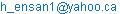Hadi Ensan. Possibility of Existence of Two Planets Between Mars and Jupiter Orbits Long Time Ago. American Journal of Astronomy and Astrophysics. Vol. 4, No. 3, 2016, pp. 34-37. doi: 10.11648/j.ajaa.20160403.11

Received: May 27, 2016; Accepted: June 15, 2016; Published: July 15, 2016

Abstract: This paper is the theory of existence of two planets in long time ago between the orbits of Mars and Jupiter. As it is shown here the planets rate of change of their angular velocity in the orbits circulating around Sun is between two numbers less than one. Although this number is not an exact number but Jupiter’s number is out of this domain. Also when we look at the distances of the planets from Sun, we see a big gap of orbital distance between Mars and Jupiter. For explanation of such irregularity of distances and also angular velocity, especially for planet of Jupiter, Two rates of changes are introduced here. First is the rate of change of distances for planets and the second is the rate of change of angular velocities for them. By calculating those average values and comparison with the values for planet of Jupiter, we could conclude the possibility that could had existed not one, but two planets between Mars and Jupiter. Perhaps by asteroids collisions and then by gravity of Jupiter they have been destructed. Now, there exists Asteroids Belt between the orbits of Mars and Jupiter. The paper explains this possibility of existence of such planets and their approximate distances from the sun and their orbital angular velocities that they could have had. By calculation mentioned in this paper the approximate medium orbit distance of the first planet (X1) from sun could had been: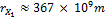and its average angular velocity: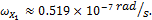For the second planet (X2), the approximate medium orbit distance from sun could had been: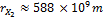and its average angular velocity: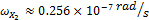.

Keywords: Two More Planets, Distance Gap, Angular Velocity Gap

Contents

1. Introduction

In our solar system several physical quantities or statistics for planets and also their orbit statistics have been known Nicholas Copernicus (1473-1543) he became the first modern proponent of the heliocentric concept for moving Earth . Galilei (1610) was the first person who uses the telescope for astronomy. He discovers the large moons of Jupiter, etc.  and Kepler finds (1609) that the Mars orbit is elliptical. He proposes (1618-1621) the three planetary motion laws; also outside of solar system many planets have been discovered [2, 3, 4, 5] and the asteroids are being studied .

The planets distances from closest and farthest to Sun and their rotation period around Sun are also indicated in our solar system. Looking at the planets distance numbers (Table 1, column 3); we see the gap of unfilled orbit between the planets of Mars and Jupiter [5, 7, 9]. When we check the angular velocity of the planets in our solar system, again we consider the possibility of missing something in that region. Therefore, this question arises that:

Was there a planet or planets in ancient era? This theory wants to find out about the possibility of existence of planet or planets between Mars and Jupiter orbits in long time ago.

2. Procedure

The theory is based on the rate of change of the distances and also the rate of change of angular velocities of the planets and their average values from Sun as below:

1.  Reviewing the distances of planets from sun in our solar system (Table 1) shows that:

The Ratio of distances or rate of change (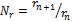,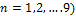that is shown here; is between 1.314 to 2.004. The distances of all planets circulating around Sun are already having been known . The distance of Jupiter from Mars is one exception that its rate change is:(1)

2.  Calculating the angular velocities of all planets from their period of circulation around sun and this fact that we already know the more distances from sun results the less angular velocity for planet. This is the result of balancing between the gravity for and the centripetal force :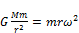(2)

This equation yields: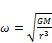(3)

This shows that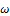or angular velocity for each planet is independent of its mass and has reverse relation to its root of cubic distant from Sun. Where; G is the Gravitational constant and M is mass of Sun. Although the orbits of planets circulating around the Sun are not full circle, but elliptical and according to Third Kepler’, the less distance to Sun for planets results the more velocity for them when rotating on elliptical orbits.

In the Table 1 based on the existing knowledge of planets , first we calculate the period time for rotating all planets around Sun (column 4) in SI system, then we calculate their angular velocity in unit of radian per second (column 5).

3.  Comparing them in the Table 1 for those calculations. For showing the rate of change of angular velocity for planets, it is introduced the ratio of angular velocity (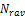) as the rate change of angular velocity for each planet respect with to angular velocity of one before planet.

The column of 6 is prepared for the ratio of angular velocities of planets or their rate of change: (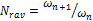,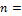1, 2, 3…9) that is between 0.3491 for Venus and 0.6628 for Pluto. This number for Jupiter is 0.1590, which is out of that range. The rate of change of angular velocity for Jupiter respect with to Mars is an exception.

Table 1. The ordinary numbers are related to the existing planets and the italicized numbers and letters belong to the era when two destructed planets were between the orbits of Mars and Jupiter.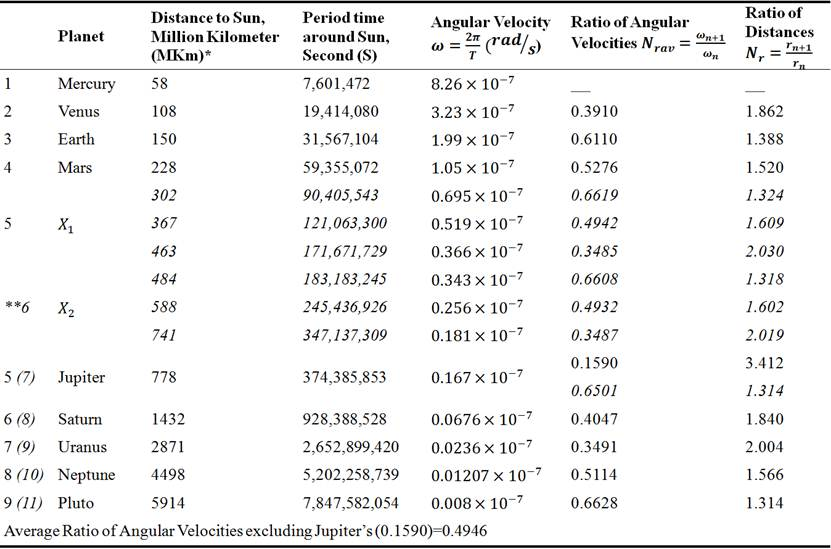All the numbers are calculated by author except the numbers in column indicted by *. *This column is referred to .

**The numbers indicated for column 6 are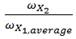and for column 7 are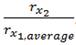.

The big empty distance or unfilled orbits between Mars and Jupiter and also very low rate of change of Jupiter angular velocity respect with to Mars leads us to guess that there had existed planets in ancient era.

4.  Assuming the two numbers that we have now could be used for finding the physical quantity or quantities of the planets that perhaps they had existed. If it or they had existed, then it or they should have had these conditions:

5.  Their Rate of Change of Distance or Distances;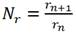should be in the range of all other planets.

6.  Their Rate of Change of Angular Velocity or Portion Angular Velocity;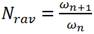should be in the range of all other planets.

7.  How can conclude that two planets had existed between the orbits of Mars and Jupiter?

Let’s look at the column 6 of table 1. The domain of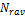is between 0.3491 to 0.6628 with one exception for Jupiter (0.1590). The average amount ofexcluding Jupiter’s is 0.4946.

If we accept that the average ratio of angular velocities of planets () could be a Physics fact (not introduced or proven so far, this the first time that it was shown here, but big gap of distance and irregularity of angular velocity between Mars and Jupiter could be a positive sign for it to find the solution), then we can substitutenumber for Jupiter at the time of existing the planet of X.

Angular velocity of that planet (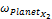) will be found as below:for Jupiter planet=0.1590(4)

If there had existed a planet between Jupiter and Mars then by using (2) we can get its angular velocity. It is named the planet of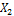. Why is it indicated the index of 2? The reason is, as we will see the single planet could not satisfy the irregularities of distances and also angular velocities. Therefore, existence of second planet is needed for solving the irregularities. The calculations could be as below: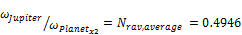(5)

based on the existing knowledge for Jupiter (table 1)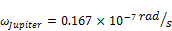(6)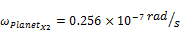(7)

Now, dividing (7) to angular velocity of Mars using table 1 yields us: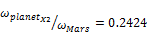(8)

This number is smaller than The Average Ratio of Angular Velocities Number (ARAVN) or (0.4946). Therefore, there could had existed another planet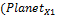) between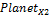and Mars and now we examine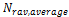for this planet.(9)

By using angular velocity of Mars (table 1), we will have: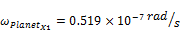(10)

We have reached to this point that based on, and entering two planets to the orbits between Mars and Jupiter the irregularity of angular velocities and also big gap of empty distance could solve. Now, we calculate the distances of such planets from Sun.

By having angular velocity for planets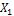and, (7) and (10), we can calculate other statistics like their circulating period and distances to sun. Equation between centripetal force and gravitation force  gives us the radius of orbit:(11)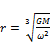(12)

where G is gravitational constant and M is the mass of Sun,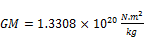(13)(14)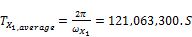(15)

continuing the same for planet of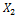, yields us: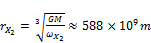(16)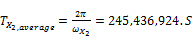(17)

By substituting the minimum and maximum amount of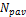(0.3491 for Venus and 0.6628 for Pluto) in (5) and (9) yields the domain for angular velocities and distances of Planetsand. Therefore, the domain for angular velocity ofis between the two below numbers: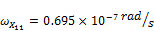(18)(19)

and the domain for its distances are: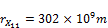(20)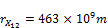(21)

The procedure should follow for the planet of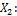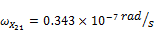(22)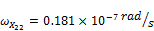(23)

and for its domain of distance to Sun, We will have: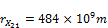(24)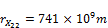(25)

8.  As we see now, the assumption of: The Possibility of Existence of Two Planets between Mars and Jupiter Orbits Long Time Ago yields us that at the time of existence of them:

a.  There was not big gap or irregularity of distances between Mars and Jupiter, because the rate of change of distances instead of existing number of 3.412 (out of range), will be change to 1.323 (in the range).

b.  There was not irregularity of angular velocity between Mars and Jupiter, because the rate of change of the Angular Velocity from existing number of 0.1590 (out of range), will be changed to 0.6501 (in the range).

9.  Like all other theories in sciences and physic, this theory also needs observations and experiments.

At the table the italicized numbers and letters belong to the era that two planets had existed between the orbits of Mars and Jupiter.

3. Summery and Conclusion

There is big gap of distance between Mars and Jupiter and also when we calculate the angular velocity and compare them together, we see deep change of angular velocity for Jupiter. This brings us this question why those parameters for planet of Jupiter have such differences. For describing of these irregularities in this paper, it was introduced two numbers. First is the ratio of distances of planets from Sun (), and the second that is more important is the Ratio of Angular Velocity (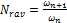). By calculating these numbers and getting their average and comparing them and continuing, we can reach the conclusion that there is possibility of existence of two planets between the orbits of Mars and Jupiter long time ago.

References

1. J. K. Wagner, Introduction to THE SOLAR SYSTEM, (Saunders College Publishing, USA, 1991) p23
2. F. J. Bueche, INTRODUCTION TO PHYSICS FOR SCIENTISTS AND ENGINEERS, 4th edition (McGraw-Hill International Editions, Singapore, 1986) p46
3. M. A. Garlick, ASTRONOMY, A visual guide, (Firefly Books Ltd. 2004), p18-19 and p 74-75
4. M. Woolfson, The Formation of the Solar System, Theories old and New (Imperial College Press, U.K., 2015) p70
5. E. Uchupi, K. Emery, Morphology of the Rocky Members of The Solar System, (Spinger-Verlag, Germany, 1993) p260
6. B. W. Jones, The Solar System, The Open University, (Pergamum Press, Great Britain, 1984) p96
7. K, Batygin et al., Jupiter’s decisive role in the inner Solar System’s early evolution, Department of Astronomy & Astrophysics, University of California, USA, vol. 112, no. 14, April 7, 2015) p4214-4217 http://www.pnas.org/content/112/14/4214.full.pdf?sid=5d569be8-7d51-43b8-bdc5-b8ad4ffeafc9
8. F. J. Bueche, Principle of Physics, 5th edition (McGraw-Hill International Editions, Singapore, 1988) p146-147, and p151
9. J. Horner et al., The role of Jupiter in driving Earth’s orbital Evolution, (University of New South Wales, Australia, et al. 2013) p1-3 .https://arxiv.org/ftp/arxiv/papers/1401/1401.6741.pdf
10. D. Jewitt1 et al. Hubble Space Telescope Observations ofActive Asteroid 324P/La Sagra, (University of California) p2-3 http://www2.ess.ucla.edu/~jewitt/papers/2016/JAW16.pdf

 Contents 1. 2. 3.
Article ToolsAbstractPDF(193K)